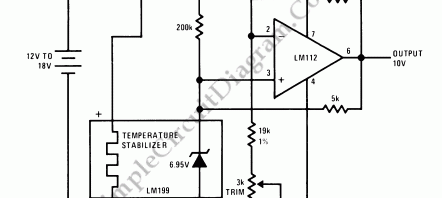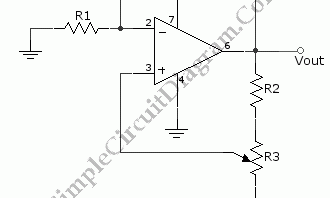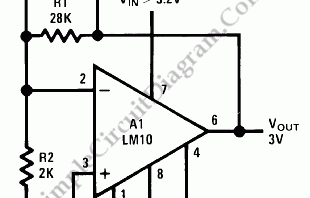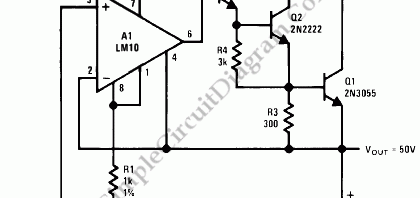## Portable Calibrator: Stable 10V ReferenceA stable voltage source is needed in many calibration process. Most electronic devices are affected by its surrounding temperature. To control all the components temperature by manipulating the surrounding temperature is not easy, but fortunately we can control only the most critical component to produce a stable voltage. The most critical component in a voltage reference is the reference diode, […]

## Variable Voltage ReferenceA positive variable voltage reference circuit is shown in the schematic diagram below. A voltage reference can be made using a zener diode, but this method produce a fix reference value. To make a variable reference, we can amplify the fix voltage reference using active components (op-amp or transistors). Here is the  schematic diagram of the circuit: This voltage reference […]

## Stabilized 3V Voltage Source/ReferenceThis LM10 circuit can be used as low voltage low current voltage source or reference. This circuit regulate the voltage from the power supply to give a very stable output. The  minimum voltage level for powering this circuit require only 0.2V above the output level, showing the low drop out  (LDO) feature of this circuit. [Circuit’s schematic diagram source: National […]